## ADTs

An Abstract Data Type (ADT) is defined only by its operations, not by its implementation.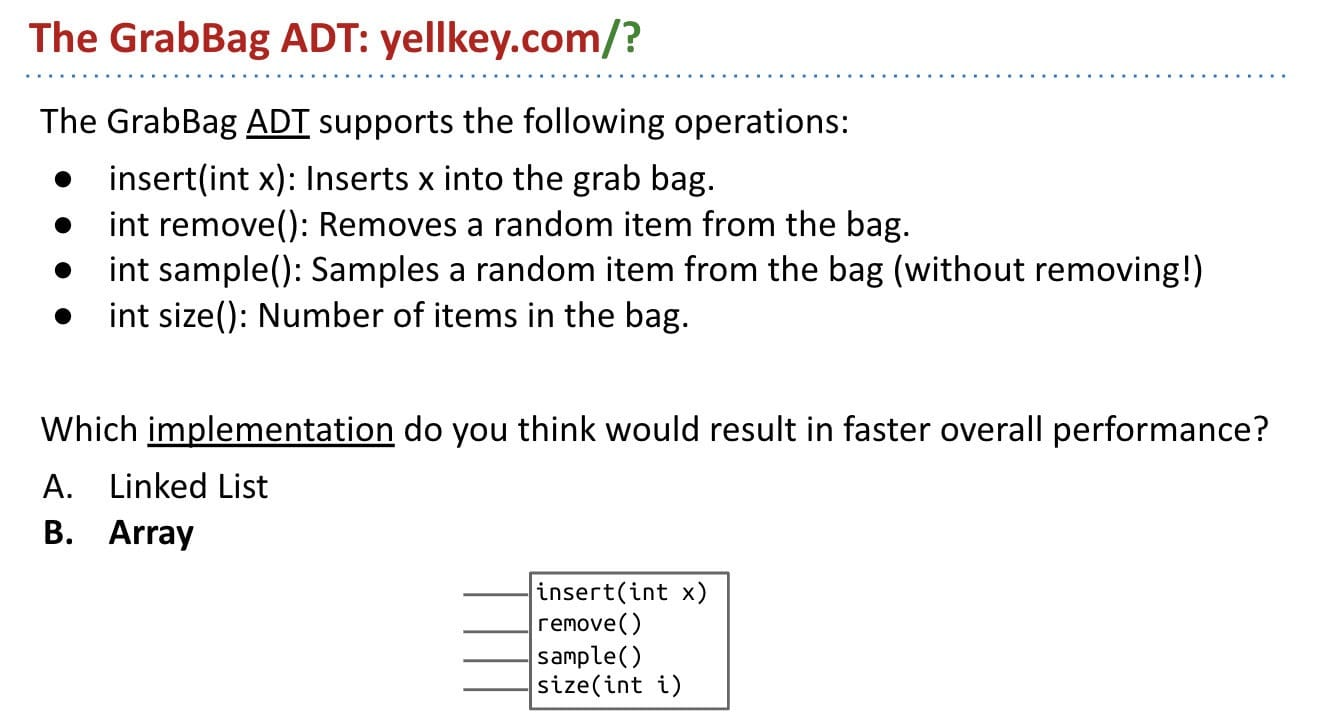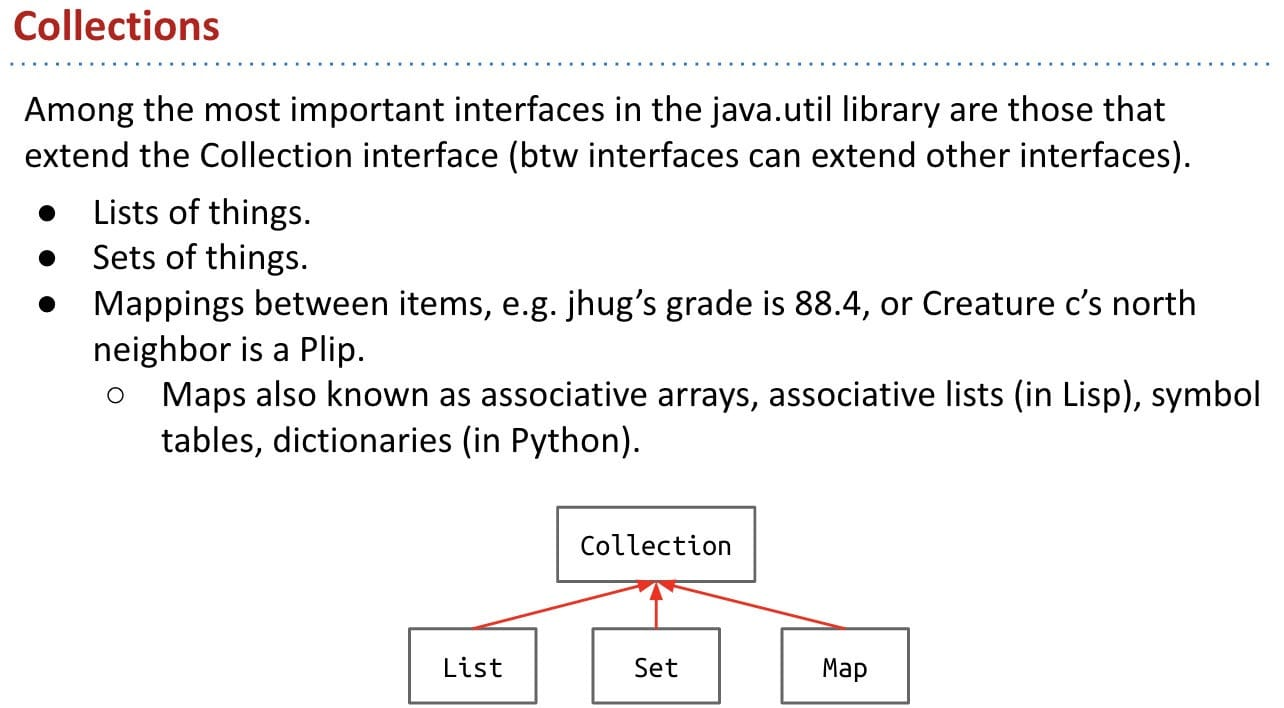## BST

Binary Search Trees

### Basics of Tree

A tree consists of:

• A set of nodes
• A set of edges that connect nodes
• Constraint: There is exactly one path (may contain several edges) between any two nodes.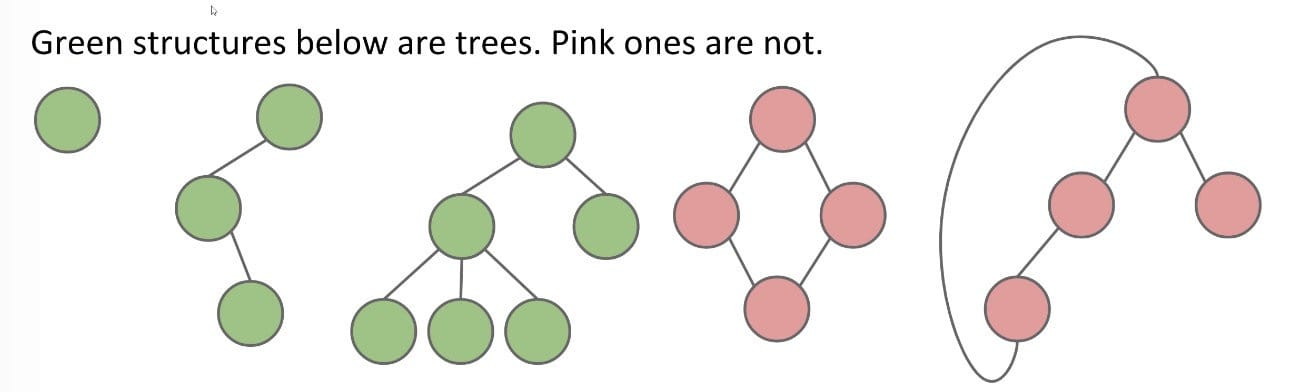In a rooted Rooted Tree, we call one node the root.

• Every node N except the root has exactly one parent, defined as the first node on the path from N to the root.
• A node with no child is called a leaf.
• Binary Tree: Every node has either 0, 1, or 2 children (subtrees).

### Definition (BST)

The BST is one of the most important ideas that you’ll ever learn in all of computer science.

Can think of the BST as representing a Set or even a Map.

Linked Lists are great, but it takes a long time to search for an item, even if the list is sorted! If the item is at the end of the list, that would take linear time.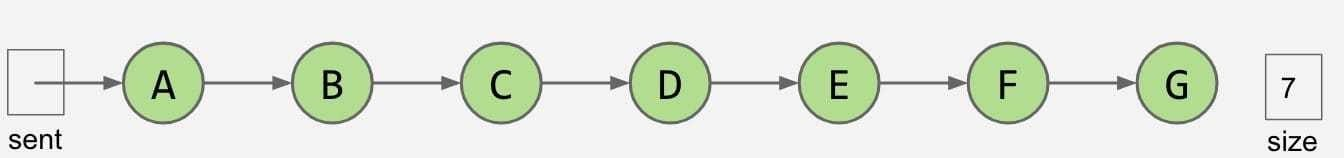Fundamental Problem: Slow search, even though it’s in order.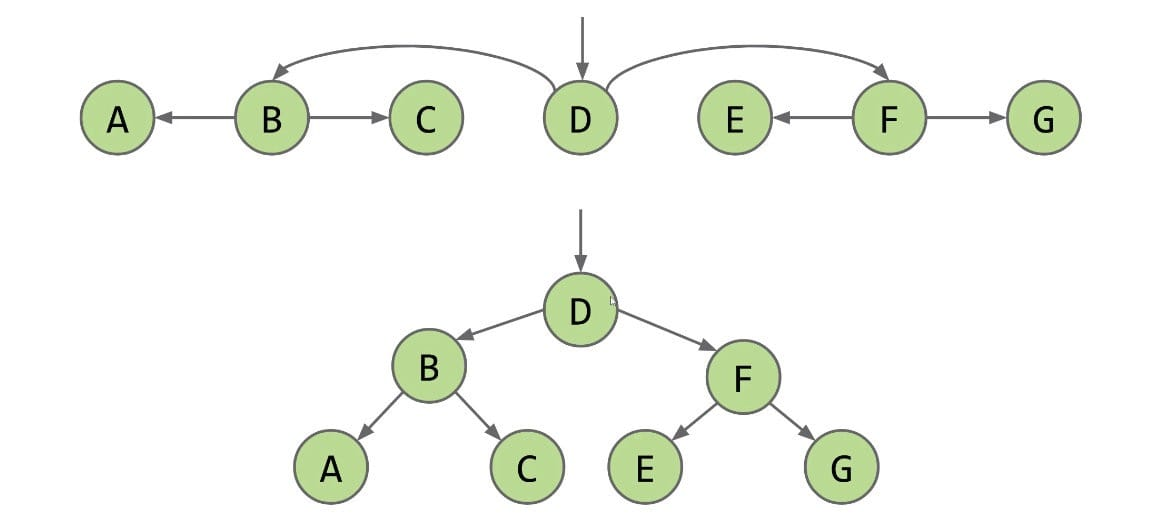Definition: A binary search tree is a rooted binary tree with the BST property.

• Every key in the left subtree is less than X’s key.
• Every key in the right subtree is greater than X’s key.

Ordering

Ordering must be complete, transitive, and antisymmetric. Given keys $p$ and $q$:

• Exactly one of $p \prec q$ and $q \prec p$ is true (not both).
• $p \prec q$ and $q \prec r$ imply $p \prec r$. (transitive)

What about an example of not complete: Family tree. Compared to your parents, you are “less than”; but compared to your cousins, there is no answer and it’s not a complete thing (in different branches).

One consequence of these rules: No duplicate keys allowed!

It keeps things simple. Most real world implementations follow this rule.

For a BST that is bushy (short and fat), we can search in $O(\log N)$ time where N is the number of nodes. For a BST that is spindly (tall and skinny), our search will take $O(N)$ time.

### Operations

$\Theta(\log N)$, Height of the tree is $\sim \log N$

#### Insert

Search for key:

• If found, do nothing
• If not found:
• Create new node
• Set appropriate link

We always insert at a leaf node!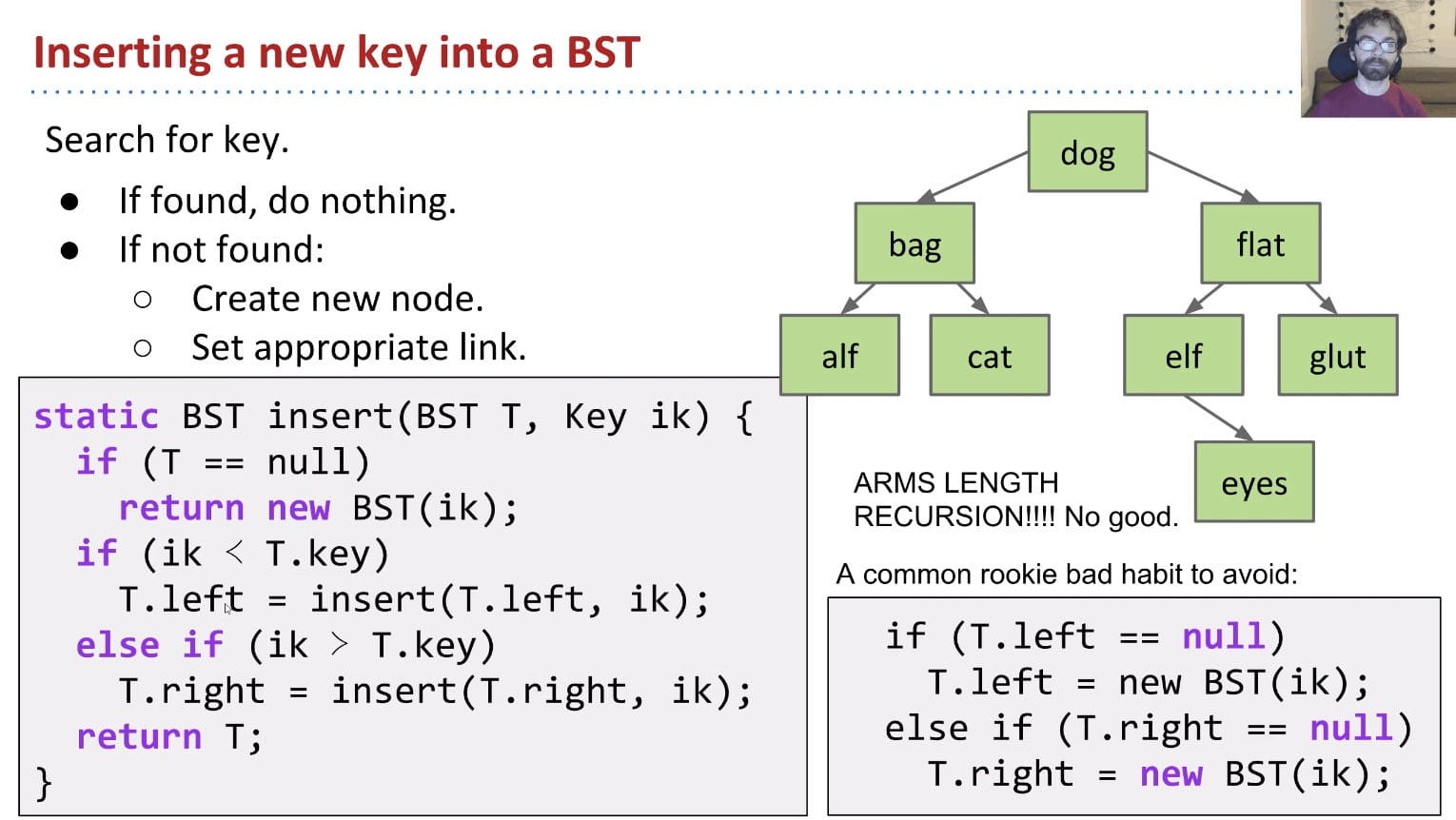My Code (not good):

#### Delete

3 Cases:

• Case 1: No children

Just sever the parent’s link, and the deleted node will be garbage collected.

• Case 2: Has 1 child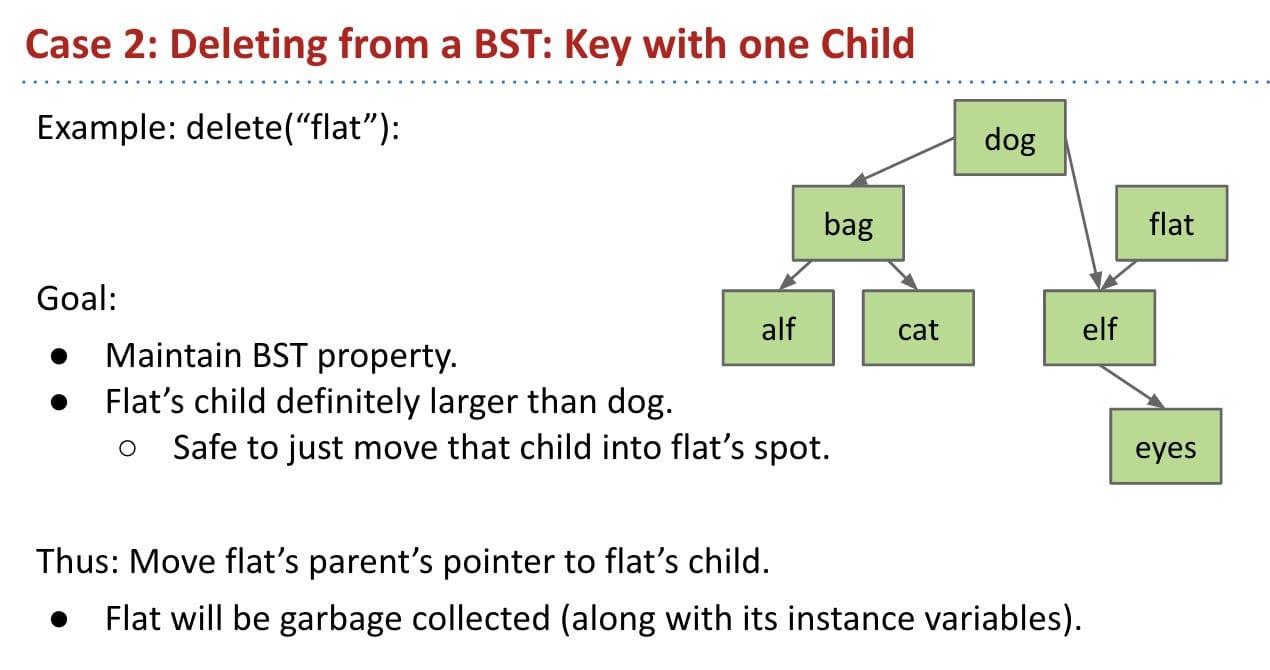We can use recursion to avoid pointer backup.

What about deleting the root?

• Case 3: Has 2 children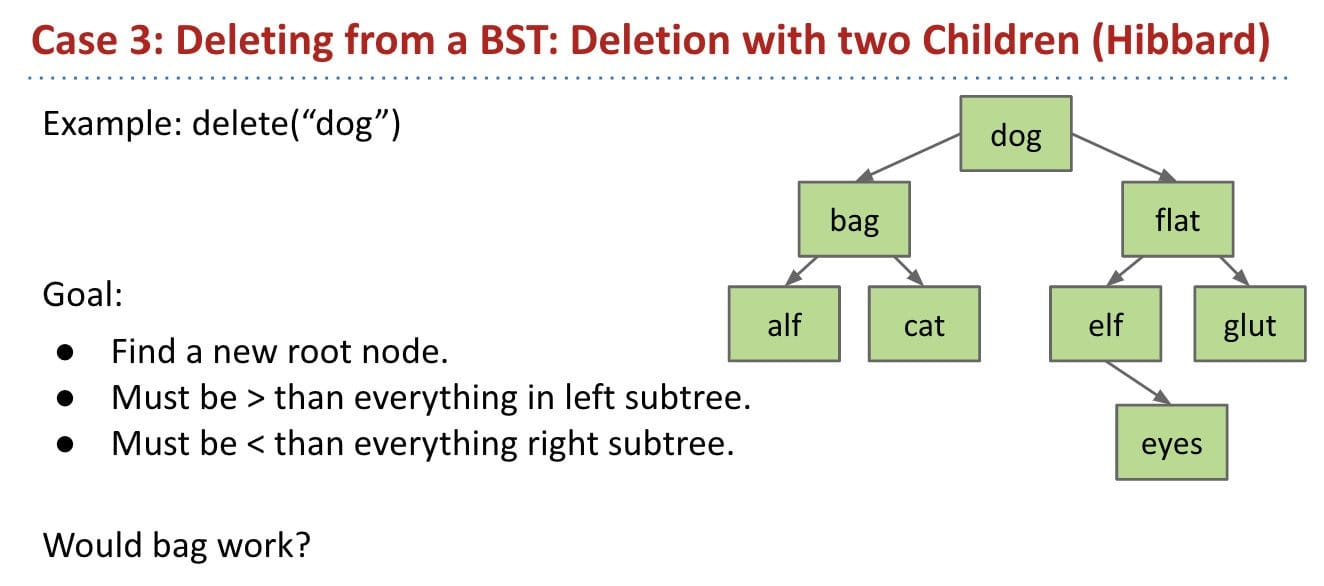No. Moving bag is not a good idea.

We can choose either predecessor "cat" or successor "elf".

In other words, cat is the largest node in the left tree; elf is the smallest node in the right tree.

• Delete cat or elf, and stick new copy in the root position: This deletion guaranteed to be either case 1 or 2 (no two children). Why?

This method is known as Hibbard deletion.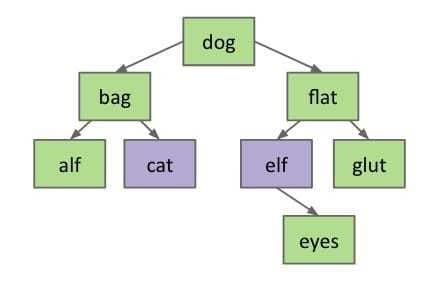For example, delete dog and move elf to the root; since we need to delete elf either, eyes will be the left child of flat (case 1).

#### Lab 7 (BSTMap)

Lab 7: BSTMap.java & Map61B.java
Algs: BST.java

numberOfNodes(BSTMap b)$sim \Theta(n)$ (b has n nodes)

z is a positive integer.

What is the running time (in big O notation) of mystery(b, z)?

### Ex (Discussion & Guide)

#### C Level

Give two orderings of the keys A X C S E R H that, when inserted into an empty BST, yield the best case height. Draw the tree.

In order: A C E H R S X

H - C - S - …
H - S - C - …

#### B Level

1. Suppose that a certain BST has keys that are integers between 1 and 10, and we search for 5. Which sequence(s) of keys below are possible during the search for 5?

Each time one level, no backward

a. 10, 9, 8, 7, 6, 5 (o)
b. 4, 10, 8, 7, 5 (o)
c. 1, 10, 2, 9, 3, 8, 4, 7, 6, 5 (o) a chain
d. 2, 7, 3, 8, 4, 5 (x) 8 > 7
e. 1, 2, 10, 4, 8, 5 (o)

2. Is the delete operation commutative? In other words, if we delete x, then y, do we always get the same tree as if we delete y, then x?

Maybe yes! Couldn’t think of any case.

3. Problem 1 from the Fall 2014 midterm #2.

• Either 0 or 2 not-null children (complete tree) - $O(\log N)$
• Either 0 or 2 children - $O(N)$
• Either 0 or 1 child - $O(N)$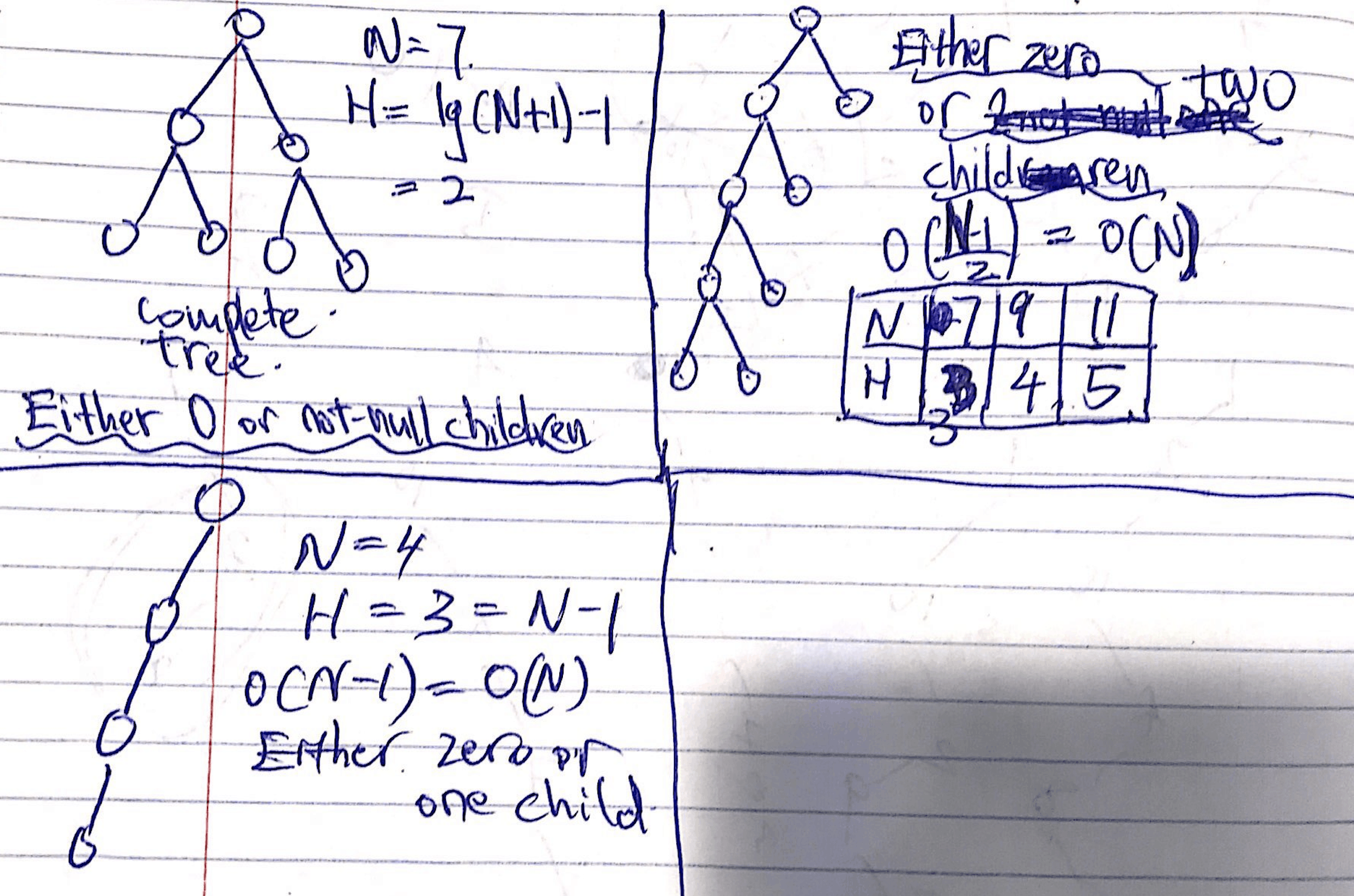#### A+ Level

Problem 3 from the Fall 2009 midterm.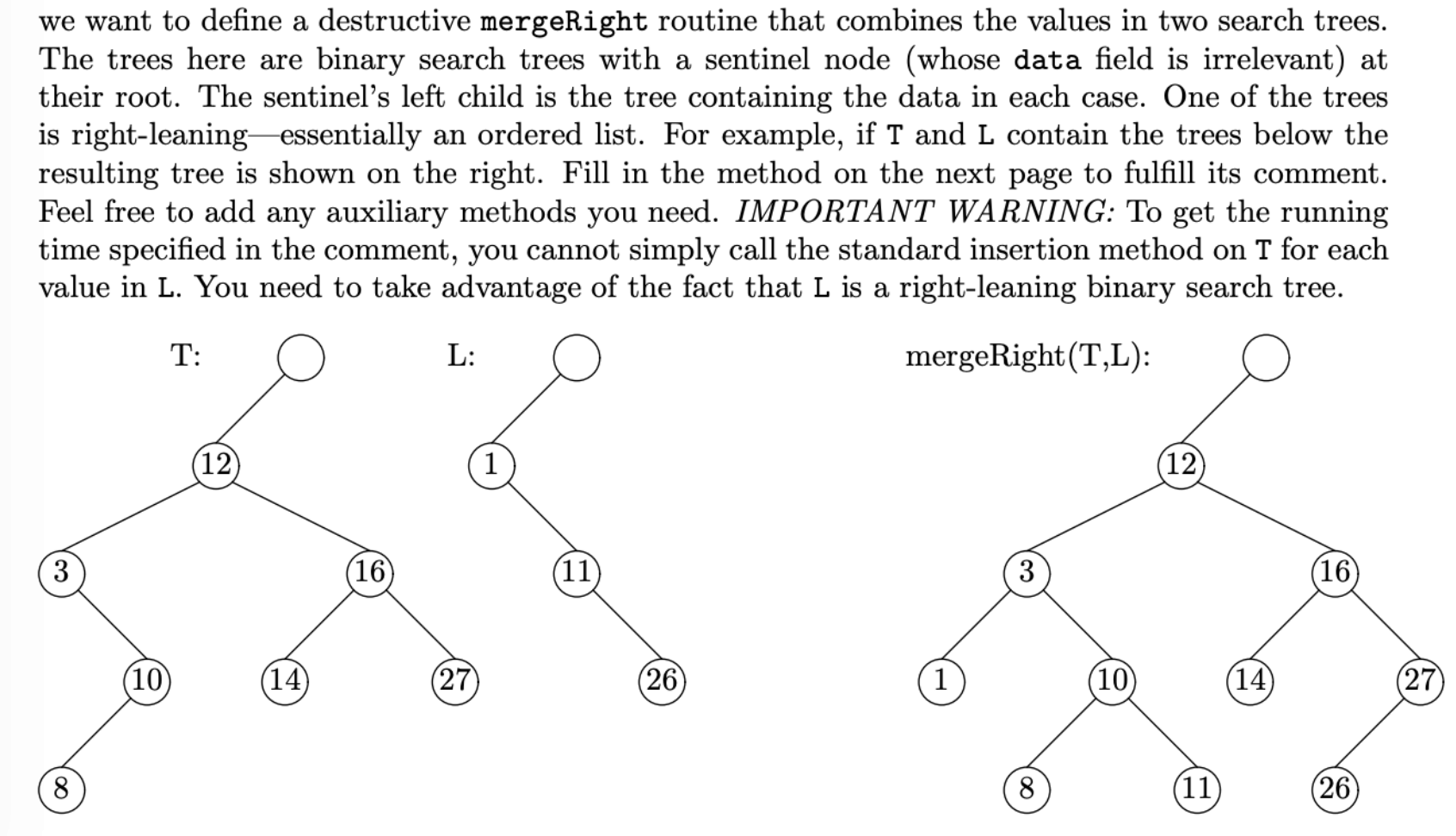I think the worst case is $O(L \log{T})$

#### Is This a BST?

From Discussion 7, Spring 2019

Buggy Version:

// 5
// / \
// 3 8
// / \ / \
// 1 6 7 9 (6 is larger than 5)

Bug-free Version:

## Challenge Lab 7

Challenge Lab 7: link
Code: link

The Internal Path Length of a BST is defined as the average depth times the number of nodes. Or equivalently, it is the sum of the lengths of the paths to every node. The internal and external path lengths are related by:

where $n$ is the number of internal nodes.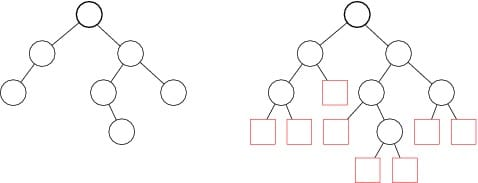Implementation of OIPL: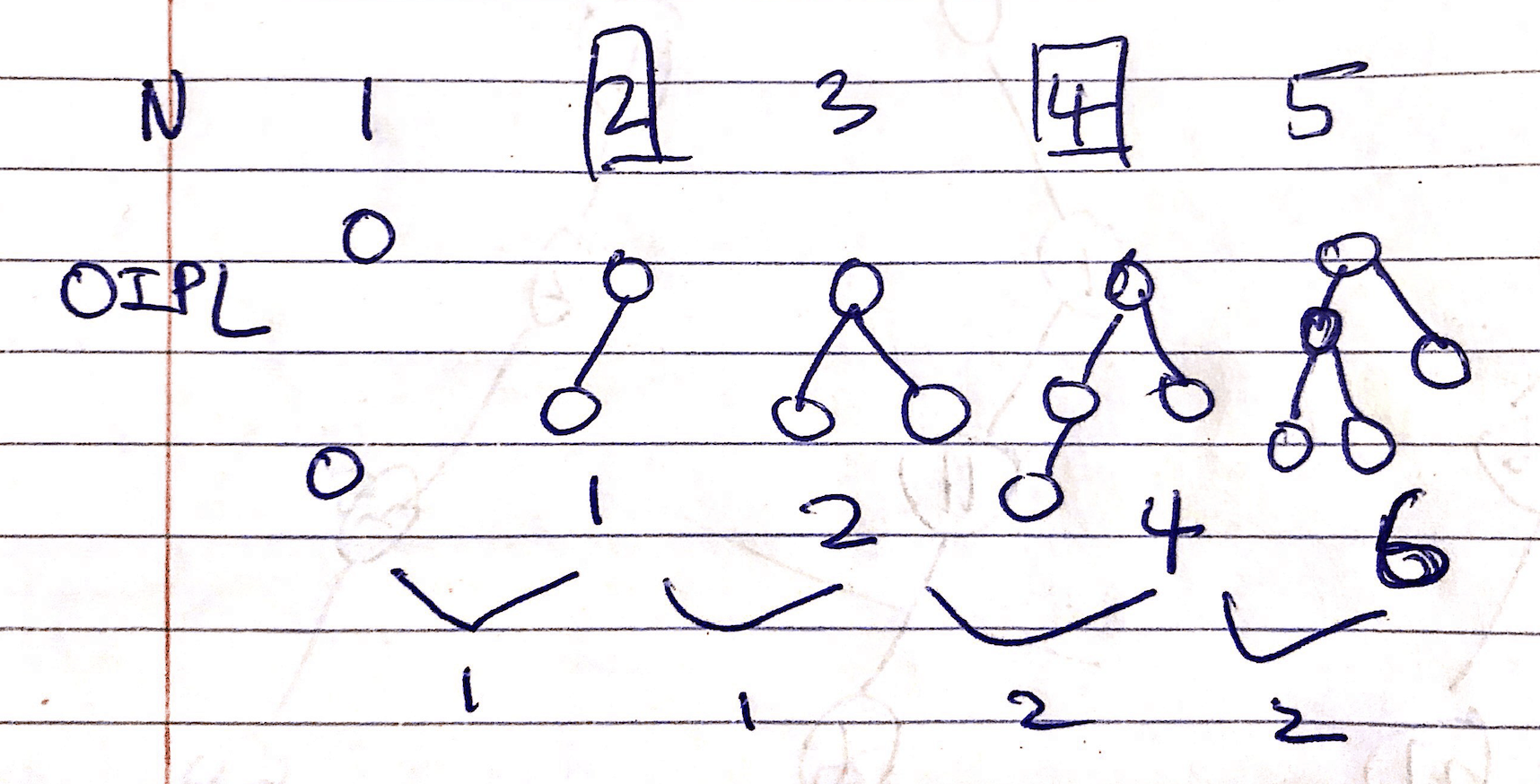Reference: Research Problem about Average Depth

Randomly picking between successor and predecessor will result in 88% of the starting depth. Nobody knows why this happens.

0%